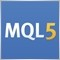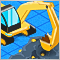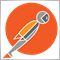# Libraries: CDouble & CDoubleVector151559

A library for common rounding methods used in MQL development, primitive wrapper class for type (double), and vector for CDouble objects. MQL5 and MQL4 compatible!

### CDouble

The CDouble class wraps a value of the primitive type double in an object. In addition, this class provides several methods and static methods for rounding doubles and arrays/collections of type double, as well as converting doubles to other data types.

Declaration

`class CDouble : public CObject`

Title

`#include <Double.mqh>`

Inheritance hierarchy

Virtual Methods implemented/overridden from class CObject: Type, Load, Save, Compare.

### CDoubleVector

The CDoubleVector class is an object pointer collection specialized for CDouble dynamic instances.

Declaration

`class CDoubleVector : public CArrayObj`

Title

`#include <Double.mqh>`

Inheritance hierarchy

Virtual Methods implemented/overridden from class CArrayObj: CreateElement.

Author: nicholishen15361

Try to remove the constraint

Note: Only one arithmetic operator can be used per statement.

And try to make comparison operators "static".2391

fxsaber:

Try to remove the constraint

And try to make comparison operators "static".

Operators cannot be declared 'static'.

There can be more than one (overload) arithmetic operation per statement provided that each set of two operands are wrapped in parenthesis in the correct order. I still don't recommend this.

```CDouble foo = 3, bar = 4, spam = 3;

CDouble error   = foo+bar+spam; //ERROR

CDouble error_too = (pi2 + pi5)+pi2; //ERROR
CDouble correct = foo+(bar+spam);// OK!

```2391

In order to clear up any possible confusion, the proper way to handle arithmetic for statements with more than one operator is to not use the overloaded operators, but instead use one of the applicable value get methods.

```CDouble sum, foo=3, bar=2, spam=1;
sum = foo.AsRawDouble() + bar.AsRounded() + spam.AsRoundedTick();
Print(sum.ToString());
//6```15361

nicholishen:

Operators cannot be declared 'static'.

There can be more than one (overload) arithmetic operation per statement provided that each set of two operands are wrapped in parenthesis in the correct order. I still don't recommend this.

```class CDouble2 : public CDouble
{
private:
static CDouble2 TmpDouble;

public:
const CDouble2* const operator +( const double Value ) const
{
CDouble2::TmpDouble = this.m_value + Value;

return(&CDouble2::TmpDouble);
}

const CDouble2* const operator +( const CDouble2 &Other ) const
{
return(this + Other.m_value);
}

static CDouble2* const Compare2( const double Value )
{
CDouble2::TmpDouble = Value;

return(&CDouble2::TmpDouble);
}

static CDouble2* const Compare2( const CDouble2 &Other )
{
CDouble2::TmpDouble = Other;

return(&CDouble2::TmpDouble);
}
};

static CDouble2 CDouble2::TmpDouble;

#define _CP(A) CDouble2::Compare2(A)

#define PRINT(A) Print(#A + " = " + (string)(A));

void OnStart()
{
CDouble2 foo = 3, bar = 4, spam = 3;
CDouble2 error   = foo+bar+spam + foo+bar+spam; //OK!

PRINT(error.ToString()); // 10
PRINT(_CP(foo + error + 5) > 2);
PRINT(_CP(25) > foo + bar + 7 +spam);
PRINT((foo + bar + spam + 9).ToString());
PRINT((_CP(9) + foo).ToString());
PRINT(foo + 7 > 11)
}```

Result

```error.ToString() = 20
_CP(foo+error+5)>2 = true
_CP(25)>foo+bar+7+spam = false
(foo+bar+spam+9).ToString() = 19
(_CP(9)+foo).ToString() = 12
foo+7>11 = false```2391

fxsaber:

Result

This is very clever and I like it a lot, but it's too clever for most users... (we both have been accused of that in the forums ;) I'll commit your changes to my personal library, and others can as well, but for the benefit of the larger base of users I'm going to keep it simple and stick with the official recommendation of calling one of the getter methods. ( eg. num1.AsRounded() * num2.AsRounded() + num3.AsRounded() )

FWIW I personally like (num1*num2+num3).AsRounded()

Challenges with CDouble2 as proposed:

```void Func(double param)
{
}

void OnStart()
{
CDouble2 foo = 2, bar = 3;
double number = foo+bar; //ERROR
Func(foo+bar); //ERROR
}```2391

### * Version 1.01:

• Fixed bug where arithmetic operators were not returning rounded values.
• Added symbol setter method to set the symbol after the constructor has been called1695

Hello, nicholishen. I have tested your library for some time. It is a great one and it makes rounding of prices and lots an easy job .

But, I have some concerns regarding the accuracy of your rounding methods. I found a lot of rounding errors in the functions RoundToStep(), RoundToStepUp(), RoundToStepDown() and RoundToTick(). These errors always occur at edge numbers which terminate in 5 (like 1.12345).

For example CDouble::RoundToStep(1.700605, 0.00001) returns 1.70060, instead of the correct result 1.70061

The equation round(number / point) * point, should be corrected to round(number * power) / power, where both point and power are derived from the number of decimal digits you would like to round to.

Because the value of 1 point which is supposed = 0.00001, is actually encoded as 0.0000100000000000000008180305391403130954586231382563710 as a 64-bits double-precision floating point. This causes the final result from you rounding method, round(number / point) * point,  to drift from the correct result by 1 point (0.00001), very often.

In addition, in order to do a proper 'arithmetic' rounding (Midpoint Rounding away from zero), a good method is to add or subtract a half-epsilon as a correction. (This will offset any half-to-even rounding that has been applied by the processor, as mandated by the IEEE-754 specs, particularly at the midpoint edge cases).

The mql's NormalizeDouble() function handles all those issues correctly, you should use it to do proper 'arithmetic' rounding.

Here, also is the source code of one function I wrote to do arithmetic rounding, you can test it yourself. This function has the exact same results as NormalizeDouble(). My function runs even faster and supports a higher level of rounding precision. (MQL's NormalizeDouble() is limited to 8 decimal digits).

```/**
* MidpointRounding away from zero ('arithmetic' rounding)
* Uses a half-epsilon for correction. (This offsets IEEE-754
* half-to-even rounding that was applied at the edge cases).
*/
double RoundCorrect(double num, int precision) {
double c = 0.5 * DBL_EPSILON * num;
//      double p = MathPow(10, precision);  //slow
double p = 1; while (precision--> 0) p *= 10;
if (num < 0)
p *= -1;
return MathRound((num + c) * p) / p;
}
```1695

Also, here is a script that you can use to debug rounding accuracy in the CDouble library. I hope you find it useful for you.

```#property strict

#define PRINT(A) Print(#A + " = ", (A))
#define forEach(element, array)  for (int __i = 0, __max = ArraySize((array)); __i < __max && ((element) = array[__i]) == (element); __i++)

#include "CDouble.mqh"

//+------------------------------------------------------------------+
//|                                                                  |
//+------------------------------------------------------------------+
string DoubleToFixed(double number, int decimals = 55) {

return StringFormat(StringFormat("%%#.%if", decimals), number);
}

//+------------------------------------------------------------------+
//|                                                                  |
//+------------------------------------------------------------------+
void OnStart() {

// test rounding of some edge cases
double numbers_3[] = {1.005, 2.175, 5.015, 16.025};
double numbers_6[] = {1.011885, 1.113325, 1.143355, 1.700605};

double num;

forEach (num, numbers_3) {

Print("----------------------------------------------");
PRINT( num );
// compare 3 functions (round to 2 digits)
PRINT( CDouble::RoundToStep(num, 0.01) );
PRINT( NormalizeDouble(num, 2) );
PRINT( RoundCorrect(num, 2) );
}

forEach (num, numbers_6) {

Print("----------------------------------------------");
PRINT( num );
// compare 3 functions (round to 5 digits)
PRINT( CDouble::RoundToStep(num, 0.00001) );
PRINT( NormalizeDouble(num, 5) );
PRINT( RoundCorrect(num, 5) );
}

// The cause of rounding problems in CDouble library
Print("----------------------------------------------");

PRINT( DoubleToFixed(0.01, 55) );      // 0.0000100000000000000008180305391403130954586231382563710
PRINT( DoubleToFixed(0.00001, 55) );   // 0.0100000000000000002081668171172168513294309377670288086

// compare NormalizeDouble and RoundCorrect by exact equality
Print("----------------------------------------------");

PRINT( NormalizeDouble(numbers_6, 5) == RoundCorrect(numbers_6, 5) );  // true
PRINT( NormalizeDouble(numbers_6, 4) == RoundCorrect(numbers_6, 4) );  // true
PRINT( NormalizeDouble(numbers_6, 3) == RoundCorrect(numbers_6, 3) );  // true
PRINT( NormalizeDouble(numbers_6, 2) == RoundCorrect(numbers_6, 2) );  // true
PRINT( NormalizeDouble(numbers_6, 1) == RoundCorrect(numbers_6, 1) );  // true

}

```2391

amrali:

Hello, nicholishen. I have tested your library for some time. It is a great one and it makes rounding of prices and lots an easy job .

But, I have some concerns regarding the accuracy of your rounding methods. I found a lot of rounding errors in the functions RoundToStep(), RoundToStepUp(), RoundToStepDown() and RoundToTick(). These errors always occur at edge numbers which terminate in 5 (like 1.12345).

For example CDouble::RoundToStep(1.700605, 0.00001) returns 1.70060, instead of the correct result 1.70061

The equation round(number / point) * point, should be corrected to round(number * power) / power, where both point and power are derived from the number of decimal digits you would like to round to.

Because the value of 1 point which is supposed = 0.00001, is actually encoded as 0.0000100000000000000008180305391403130954586231382563710 as a 64-bits double-precision floating point. This causes the final result from you rounding method, round(number / point) * point,  to drift from the correct result by 1 point (0.00001), very often.

In addition, in order to do a proper 'arithmetic' rounding (Midpoint Rounding away from zero), a good method is to add or subtract a half-epsilon as a correction. (This will offset any half-to-even rounding that has been applied by the processor, as mandated by the IEEE-754 specs, particularly at the midpoint edge cases).

The mql's NormalizeDouble() function handles all those issues correctly, you should use it to do proper 'arithmetic' rounding.

Here, also is the source code of one function I wrote to do arithmetic rounding, you can test it yourself. This function has the exact same results as NormalizeDouble(). My function runs even faster and supports a higher level of rounding precision. (MQL's NormalizeDouble() is limited to 8 decimal digits).

Thanks for pointing this out. I'll be updating the code to use NormalizeDouble instead of round.

`step * NormalizeDouble(number_to_round / step, 0)`38336

amrali:

Hello, nicholishen. I have tested your library for some time. It is a great one and it makes rounding of prices and lots an easy job .

But, I have some concerns regarding the accuracy of your rounding methods. I found a lot of rounding errors in the functions RoundToStep(), RoundToStepUp(), RoundToStepDown() and RoundToTick(). These errors always occur at edge numbers which terminate in 5 (like 1.12345).

For example CDouble::RoundToStep(1.700605, 0.00001) returns 1.70060, instead of the correct result 1.70061

The equation round(number / point) * point, should be corrected to round(number * power) / power, where both point and power are derived from the number of decimal digits you would like to round to.

Because the value of 1 point which is supposed = 0.00001, is actually encoded as 0.0000100000000000000008180305391403130954586231382563710 as a 64-bits double-precision floating point. This causes the final result from you rounding method, round(number / point) * point,  to drift from the correct result by 1 point (0.00001), very often.

However from a trading point of view, you need either 1.70060 or 1.70061, they are both correct. So maybe you will want to chose the best one according to your trading operation, rather than relying on a mathematical rounding scheme.